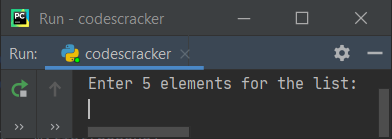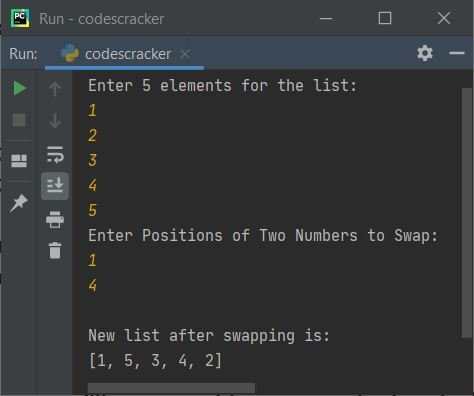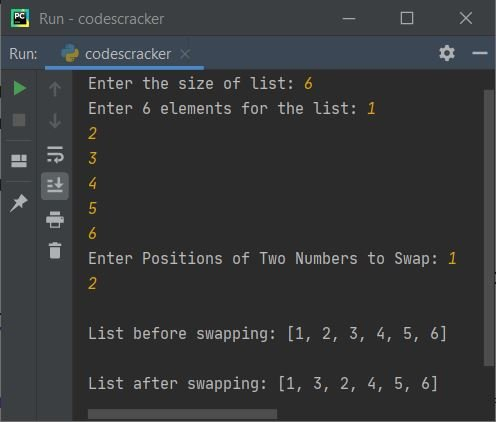# Python Program to Swap Two Elements in a List

This article deals with some programs in Python that swaps any two given elements from a given list by user at run-time. Here are the list of programs covered in this article:

• Swap two elements in a list of 5 elements
• Swap two elements in a list of n elements

Note - In all the programs given below, we've consider the position value as index value of the list. Since indexing starts with 0, therefore if user enters 1 as position after executing the program, it gets considered as 1th index element, that will actually be the second element.

But you can modify it, just by adding 1 with both the input values before applying the swapping code.

## Swap Two Elements in List

The question is, write a Python program to swap any two elements in a list. Both positions of two elements and list must be received by user at run-time. The program given below is answer to this question:

```mylist = []
print("Enter 5 elements for the list: ")
for i in range(5):
mylist.append(int(input()))

print("Enter Positions of Two Numbers to Swap: ")
posOne = int(input())
posTwo = int(input())

posOneElement = mylist[posOne]
posTwoElement = mylist[posTwo]

mylist[posOne] = posTwoElement
mylist[posTwo] = posOneElement

print(mylist)```

Here is its sample run. This is the snapshot of the initial output produced by this Python program:Now supply the input say 1, 2, 3, 4, 5 as five elements for the list, then 1 and 4 as the position (index) number for the two elements to swap. Here is its sample output after supplying exactly same inputs:Note - When considering position as index, the list suppose [1, 2, 3, 4, 5], where 1 is considered as zeroth element, 2 is considered as first element, 3 is considered as second element, and so on. Because indexing in list always starts with 0.

## Swap Two Elements in List of n Elements

This is actually the modified version of previous program. Since this program leaves the size of list to be defined by user at run-time along with its elements. Rest of the things are similar to previous program.

```mylist = []
print("Enter the size of list: ", end="")
tot = int(input())

print("Enter " +str(tot)+ " elements for the list: ", end="")
for i in range(tot):
mylist.append(int(input()))

print("Enter Positions of Two Numbers to Swap: ", end="")
posOne = int(input())
posTwo = int(input())

posOneElement = mylist[posOne]
posTwoElement = mylist[posTwo]

print("\nList before swapping: ", end="")
print(mylist)

mylist[posOne] = posTwoElement
mylist[posTwo] = posOneElement

print("\nList after swapping: ", end="")
print(mylist)```

The snapshot given below shows the final output produced by this program after providing the input, 6 as size of list, 1, 2, 3, 4, 5, 6 as its six elements, and 1 and 2 as position of elements to swap.You can also use these block of codes:

```print("\nList before swapping: ", end="")
print(mylist)

temp = mylist[posOne]
mylist[posOne] = mylist[posTwo]
mylist[posTwo] = temp```

```posOneElement = mylist[posOne]
posTwoElement = mylist[posTwo]

print("\nList before swapping: ", end="")
print(mylist)

mylist[posOne] = posTwoElement
mylist[posTwo] = posOneElement```

to do the same job as of previous program.

Python Online Test

« Previous Program Next Program »